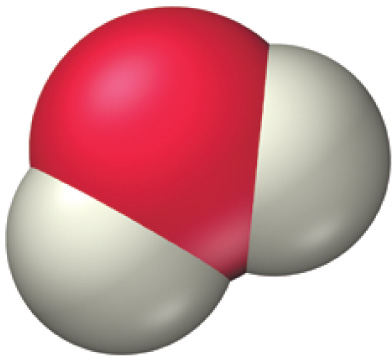# Problem: Throughout this textbook, we use space-filling molecular models to represent molecules. Which number is the best estimate for the scaling factor used in these models? In other words, by approximately what number would you have to multiply the radius of an actual oxygen atom to get the radius of the sphere used to represent the oxygen atom in the water molecule shown.

###### FREE Expert Solution

atomic radii have hundreds of picometers

spheres in space-filling molecular structures have radii centimeter

83% (246 ratings)###### Problem DetailsThroughout this textbook, we use space-filling molecular models to represent molecules. Which number is the best estimate for the scaling factor used in these models? In other words, by approximately what number would you have to multiply the radius of an actual oxygen atom to get the radius of the sphere used to represent the oxygen atom in the water molecule shown.

Frequently Asked Questions

What scientific concept do you need to know in order to solve this problem?

Our tutors have indicated that to solve this problem you will need to apply the Molecular Models concept. If you need more Molecular Models practice, you can also practice Molecular Models practice problems.

What professor is this problem relevant for?

Based on our data, we think this problem is relevant for Professor Mohammadi's class at CSULB.